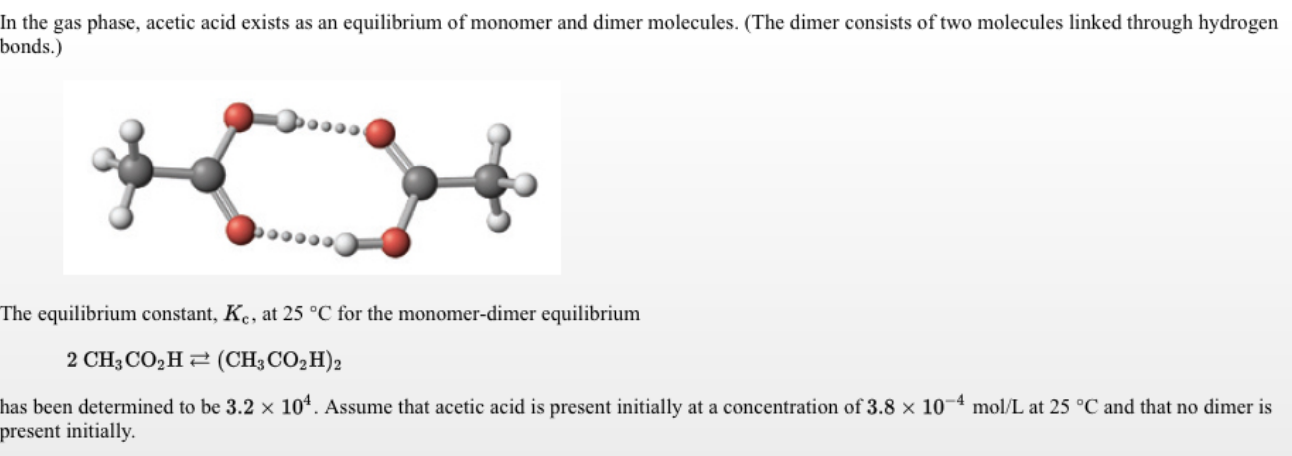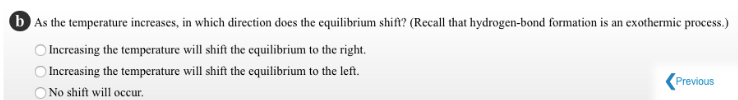# In the gas phase, acetic acid exists in an equilibrium of monomer and dimer molecules. (The dimer consists of two molecules linked through hydrogen bonds.) The equilibrium constant, Kc, at 25 degrees C for the monomer-dimer equilibrium 2 CH3CO2H ⇌ 9CH3CO2H)2 has been determined to be 3.2 x 10^4. Assume that acetic acid is present initially at a concentration of 3.8x10^-4 mol/L at 25 degrees C and that no dimer is present initially. b) As the temperature increases, in which direction does the equilibrium shift? (Recall that hydrogen-bond formation is an exothermic process.)